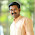## Pages

### What Is Power Factor And Its Significant

Understanding Power Factor
In order to understand this term, I am going to draw a parallel between a mechanical system and an electrical system.  When we plan to buy a new car considering the time we spend in traffic jams – one of the basic parameters we look at is the efficiency of the system.  If the manufacturer promises that the car will deliver a mileage of 18 km/Lit and we actually land up getting 15 km/ lit
we can say that the cars mileage is about 83%. This is a good method of determining how the cars combustion system operates.

>Power factor is measure of how efficiently an A.C. electrical system operates.

>Power in terms of variables is the product of VOLTAGE and CURRENT.
i.e P=VxIVoltage and current are rarely in phase. They are in phase only in ideal conditions

ϕ is the angle between Voltage and Current.

Cos ϕ is the power factor of electrical load.

Onboard ships this value is normally 0.8 lagging.
This means that the current waveform lags the voltage waveform by about 37 degrees.
Example
Let us consider the following situation: When we switch on a breaker, for eg ballast pump,
Power from the Main switch board ( P= VxI KW) is supplied to the motor.
This is called the Actual power (KW).  Actual power does useful work and overcomes normal line losses.

Reactive power (KVAR) is the volt-amperes necessary to provide MAGNETIZING POWER for INDUCTIVE LOADS.

Apparent power (KVA) is the sum of the total power required to run the motor & the reactive power.Significance: A high power factor is an indication that the apparent power requirements (KVA) is very close to the actual power requirement (KW).
This means that if the power factor is 0.9
And we supply 100 KW to the motor 90 KW is used effectively to turn the rotor and only 10 KW is lost on inductive loads and losses.  Having a high power factor is of great benefit to the consumer as well as the producer.1.